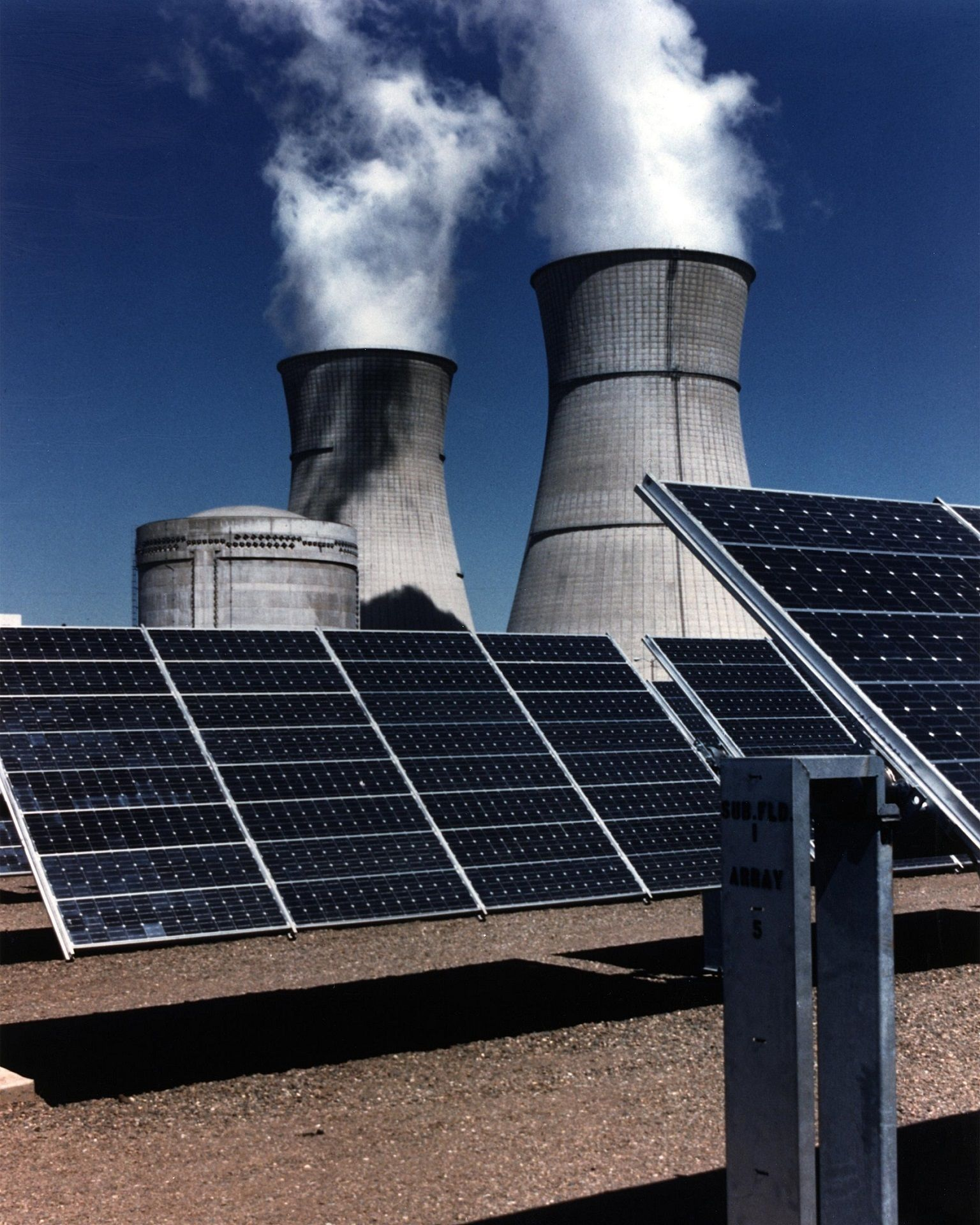# Solar Cell Part 2: Surface TemperatureHere we have a simplified version of a solar
cell, and we have right here our semiconductor silicon layer, on top of that is adhesive,
and then we have glass. And what we need to find out is the temperature of the silicon, that we're going to call T 1, as well as the temperature at the surface, that we're
going to call T 3. So since we have heat travelling through different materials and through different
modes, we can draw a thermal circuit and use the resistances to solve for our temperatures.
So we're going to use as our governing equation that q equals delta T divided by the sum of
the resistances between those two temperatures. And in our case, we're going to say T 1 minus
T infinity, where T infinity is the temperature of the air, or 25 degrees C, is going to
be the sum of the total resistances between them. So if we're trying to find T 1, that's
going to equal our q times our total resistances, plus T infinity. Let's start by looking at
a thermal circuit for the process.

We're going to start with the silicon which is at T 1.
And now going between the silicon, we have a resistance that's caused by the adhesive,
and we get to T 2, which is at the top of the adhesive. Then we have a resistance that's
caused by the glass. Then we have the temperature of the surface, and then we have two resistances
in parallel – one is caused by the radiation (and this is T surrounding) and the other
is caused by the convection (and this is T infinity).

And both T surrounding and T infinity
are 25 degrees C. So let's look at what these resistances are. The conductive resistance
is L sub A, the length of the adhesive, divided by its thermal conductivity times the area.
Here we have L sub G, divided by k sub G times the area. Here we have the resistance of the
radiation, which was given to us in the problem. And here, we have one over the heat transfer
coefficient for convection times the area. So we solved for h in a previous screencast,
so we can write this entire circuit out in terms of our total resistances. Our total
resistance is L A divided by k A times the area, plus L sub G divided by k sub G times
the area, plus one over h A plus the resistance of the radiation, and since these two are
in parallel, this is to the minus one.Let's list what we know. So let's put these into
our equation for the total resistance and solve. We get a total resistance of 0.54 kelvin
per watt. So our T 1 is going to equal our q times this total resistance plus T infinity.
So our T 1 is going to equal 352 kelvin. So now we need to find T 3. So what we do, is
we go back that our q equals delta T times the sum of the resistances between them. And
now we're going to set it up as T 1 minus T 3, and the two resistances between them
– let's go back and look at this thermal circuit – the two resistance between T 1 and T 3 are
the two conductive resistances. So this is going to equal the sum of the conductive resistances.
And now we can write that T 3 equals T 1 minus q times this R. Let's calculate what our conductive
resistances are. They're L sub A, divided by k sub A times the area, plus L sub G, divided
by k sub G times the area.

This equals 0.0286 kelvin per watt. And now we have the necessary
information that we need, and our T 3 is going to end up equaling 349 K. And this is exactly
what you would expect, the temperature of the silicon should be the highest, followed
by that surface temperature. The surface or the temperature in between the two, if you take a look at our
picture, this T 2 should be somewhere in between 352 and 349 K. And these are all greater than
the surrounding air, which is 298 K..